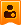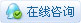##用户名 Email 自动登录 找回密码 密码 立即注册
 搜索 热搜: 眼底 图像 血管 视盘 分泌物

# opencv访问Mat图像中每个像素的值发表于 2013-3-10 21:55:54 | 显示全部楼层 |阅读模式
 以下例子源自《The OpenCV Tutorials --Release 2.4.2》2.2 How to scan images, lookup tables and time measurement with OpenCV 图像容器Mat还是先看Mat的存储形式。Mat和Matlab里的数组格式有点像，但一般是二维向量，如果是灰度图，一般存放类型；如果是RGB彩色图，存放类型。 单通道灰度图数据存放格式： 多通道的图像中，每列并列存放通道数量的子列，如RGB三通道彩色图： 注意通道的顺序反转了：BGR。通常情况内存足够大的话图像的每一行是连续存放的，也就是在内存上图像的所有数据存放成一行，这中情况在访问时可以提供很大方便。可以用 isContinuous()函数来判断图像数组是否为连续的。

x

### 相关帖子楼主| 发表于 2013-3-10 21:56:53 | 显示全部楼层
 访问图像中的像素 高效的方法：C操作符[ ]最快的是直接用C风格的内存访问操作符[]来访问： [cpp] view plaincopy Mat& ScanImageAndReduceC(Mat& I, const uchar* const table)  {      // accept only char type matrices      CV_Assert(I.depth() != sizeof(uchar));      int channels = I.channels();      int nRows = I.rows ;      int nCols = I.cols* channels;      if (I.isContinuous())      {          nCols *= nRows;          nRows = 1;      }      int i,j;      uchar* p;      for( i = 0; i < nRows; ++i)      {          p = I.ptr(i);          for ( j = 0; j < nCols; ++j)          {              p[j] = table[p[j]];          }      }      return I;  }   注意：书中这段代码是有问题的，前面写成了 [cpp] view plaincopy int nRows = I.rows * channels;  int nCols = I.cols;   一般情况 isContinous为true，运行不会出错，但你可以注释掉那个if，会有访问越界的问题。 这种访问形式就是在每行定义一个指针，然后在内存上直接连续访问。如果整个数组在内存上都是连续存放的，那么只需要定义一个指针就可以访问所有的数据！如单通道的灰度图访问方式如下： [cpp] view plaincopy uchar* p = I.data;  for( unsigned int i =0; i < ncol*nrows; ++i)      *p++ = table[*p];   安全的方法：迭代器iterator相比用指针直接访问可能出现越界问题，迭代器绝对是非常安全的方法： [cpp] view plaincopy Mat& ScanImageAndReduceIterator(Mat& I, const uchar* const table)  {      // accept only char type matrices      CV_Assert(I.depth() != sizeof(uchar));      const int channels = I.channels();      switch(channels)      {      case 1:          {              MatIterator_ it, end;              for( it = I.begin(), end = I.end(); it != end; ++it)                  *it = table[*it];              break;          }      case 3:          {              MatIterator_ it, end;              for( it = I.begin(), end = I.end(); it != end; ++it)              {                  (*it) = table[(*it)];                  (*it) = table[(*it)];                  (*it) = table[(*it)];              }          }      }      return I;  }   这里我们只定义了一个迭代器，用了一个for循环，这是因为在OpenCV里迭代器会访问每一列然后自动跳到下一行，不用管在内存上是否isContinous。另外要注意的是在三通道图像中我们定义的是 格式的迭代器，如果定义成uchar，则只能访问到B即蓝色通道的值。 这种方式虽然安全，但是挺慢的，一会儿就知道了。 更慢的方法：动态地址计算这种方法在需要连续扫描所有点的应用时并不推荐，因为它更实用与随机访问。这种方法最基本的用途是访问任意的某一行某一列： [cpp] view plaincopy Mat& ScanImageAndReduceRandomAccess(Mat& I, const uchar* const table)  {      // accept only char type matrices      CV_Assert(I.depth() != sizeof(uchar));      const int channels = I.channels();      switch(channels)      {      case 1:          {              for( int i = 0; i < I.rows; ++i)                  for( int j = 0; j < I.cols; ++j )                      I.at(i,j) = table[I.at(i,j)];              break;          }      case 3:          {              Mat_ _I = I;                for( int i = 0; i < I.rows; ++i)                  for( int j = 0; j < I.cols; ++j )                  {                      _I(i,j) = table[_I(i,j)];                      _I(i,j) = table[_I(i,j)];                      _I(i,j) = table[_I(i,j)];                  }                  I = _I;                  break;          }      }      return I;  }   因为这种方法是为随机访问设计的，所以真的是奇慢无比。。。楼主| 发表于 2013-3-10 21:58:06 | 显示全部楼层
 减小颜色空间 color space reduction现在来介绍下上述函数对每个元素的操作，也就是用table更改像素值。这里其实是做了个减小颜色空间的操作，这在一些识别之类的应用中会大大降低运算复杂度。类如uchar类型的三通道图像，每个通道取值可以是0~255，于是就有 256*256个不同的值。我们可以通过定义： 0~9 范围的像素值为 0 10~19 范围的像素值 为 10 20~29 范围的像素值为 20 。。。。。。 着这样的操作将颜色取值降低为 26*26*26 种情况。这个操作可以用一个简单的公式： 来实现，因为C++中int类型除法操作会自动截余。 类如 Iold=14; Inew=(Iold/10)*10=(14/10)*10=1*10=10; 在处理图像像素时，每个像素需要进行一遍上述计算也需要一定的时间花销。但我们注意到其实只有 0~255 种像素，即只有256种情况。进一步可以把256种计算好的结果提前存在表中 table 中，这样每种情况不需计算直接从 table 中取结果即可。 [cpp] view plaincopy int divideWith=10;   uchar table;  for (int i = 0; i < 256; ++i)      table = divideWith* (i/divideWith);   于是table存放的是值为i的像素减小颜色空间的结果，这样也就可以理解上述方法中的操作： [cpp] view plaincopy p[j] = table[p[j]];   LUT : Look up tableOpenCV 很聪明的有个 LUT 函数就是针对这种 Look up talbe 的操作： [cpp] view plaincopy Mat lookUpTable(1, 256, CV_8U);  uchar* p = lookUpTable.data;  for( int i = 0; i < 256; ++i)      p = table;  for (int i = 0; i < times; ++i)      LUT(I, lookUpTable, J);   算法计时为了验证几种方法的效率，可以用一个简单的计时和输出： [cpp] view plaincopy double t;  t = (double)getTickCount();  t = 1000*((double)getTickCount() - t)/getTickFrequency();  t /= times;   实验结果 原图： 降低颜色空间结果： 算法时间： 更清楚的时间对比表： 转载请注明出处：http://blog.csdn.net/xiaowei_cqu/article/details/7771760 实验代码下载：http://download.csdn.net/detail/xiaowei_cqu/4443761

### 本帖子中包含更多资源

x

 本版积分规则 回帖并转播 回帖后跳转到最后一页|Archiver|手机版|小黑屋|爱乐眼底图像分析 ( 京ICP备1201155号 )

GMT+8, 2021-10-21 08:58 , Processed in 0.059470 second(s), 21 queries .